# Precalculus : Circles

## Example Questions

### Example Question #17 : Determine The Equation Of A Circle In Standard Form

Write the equation for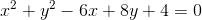in standard form

Possible Answers: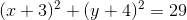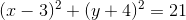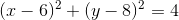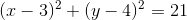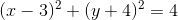Correct answer:Explanation:

To determine the standard-form equation, we'll have to complete the square for both x and y. It will be really helpful to re-group our terms to do that: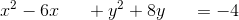Adding 9 will complete the square for x, since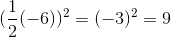Adding 16 will complete the square for y, since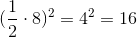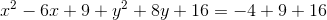Now we just need to simplify. Re-write the left side as two binomials squared, and add the numbers on the right side:### Example Question #18 : Determine The Equation Of A Circle In Standard Form

If the center of the circle is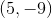and the radius is 6, what is the equation of the circle in standard form?

Possible Answers: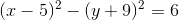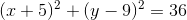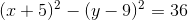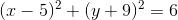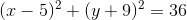Correct answer:Explanation:

Write the equation of the standard form of the circle.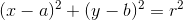where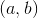is the center of the circle andis the radius.

Substitute the center and the radius into the equation.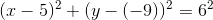Reduce this equation and leave this in standard form.### Example Question #19 : Determine The Equation Of A Circle In Standard Form

Determine the equation of a circle whose center is at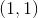and radius is.

Possible Answers: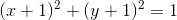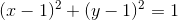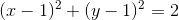Correct answer:Explanation:

To solve, simply use the formula for a circle as given below.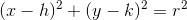Thus, our answer is:### Example Question #20 : Determine The Equation Of A Circle In Standard Form

Determine the equation for a circle in standard form, centered at (3,-4), with radius 2.

Possible Answers: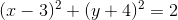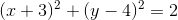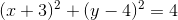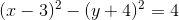Correct answer:Explanation:

Recall that the standard from for the equation of a circle iswhere (h, k) is the center, and r is the radius.  We are given the center (3, -4) and radius 2.  Therefore, h = 3, k = -4, and r = 2.  Plugging these vaules into the equation gives us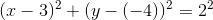### Example Question #21 : Determine The Equation Of A Circle In Standard Form

A circle centered at (6,1) passes through (11,13).  Write an equation for the circle in standard form.

Possible Answers: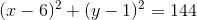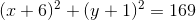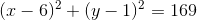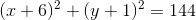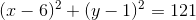Correct answer:Explanation:

Recall the equation of a circle in standard form:, where (h, k) is the center and r is the radius.

In this problem, we are given the center, but no radius.  We must use the other piece of information to find the radius.  The second point given is a point on the circle.  The definition of a radius is the distance between the center and any point on the circle.  Therefore, the radius is equal to the distance between (6,1) and (11,13).  Using the distance formula,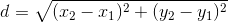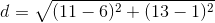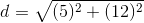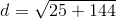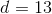Therefore, the radius is 13.  Plugging all the information into the standard form of a circle gives us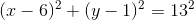### Example Question #22 : Determine The Equation Of A Circle In Standard Form

Find the equation of a circle whose center is at (0,0) and radius is 3.

Possible Answers: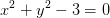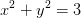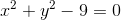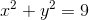Correct answer:Explanation:

To solve, simply plug in the given information into the formula for a circle.Our center is at,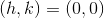and our radius is 3 therefore,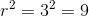Thus,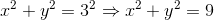### Example Question #23 : Determine The Equation Of A Circle In Standard Form

Find the equation of a circle with its vertex at the origin and a radius of 8.

Possible Answers: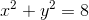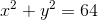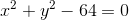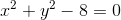Correct answer:Explanation:

To solve, simply remember standard form.

Also, the center at the origin implies the point.

Thus,### Example Question #24 : Determine The Equation Of A Circle In Standard Form

Write the equation for a circle with a center at (3,4) and a diameter of 7.

Possible Answers: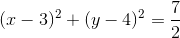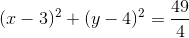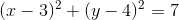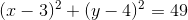Correct answer:Explanation:

The general equation for a circle is:The coordinates of the center of the circle is (h,k) and r is the radius.

The diameter is twice the radius: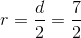So plugging in the coordinates the circle equations is: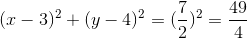### Example Question #25 : Determine The Equation Of A Circle In Standard Form

Write the equation for a circle with a center at (-2,4) and a diameter of 12.

Possible Answers: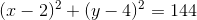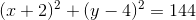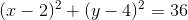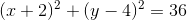Correct answer:Explanation:

The general equation for a circle is:The coordinates of the center of the circle is (h,k) and r is the radius.

The diameter is twice the radius: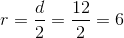So plugging in the coordinates the circle equations is: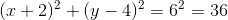### Example Question #26 : Determine The Equation Of A Circle In Standard Form

Write the equation for a circle with a center at (2,3) and a radius of 4.

Possible Answers: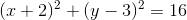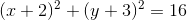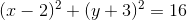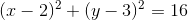Correct answer:Explanation:

The general equation for a circle is:The coordinates of the center of the circle is (h,k) and r is the radius.

So plugging in the coordinates the circle equations is: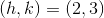and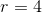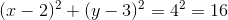### All Precalculus Resources